# Data and Functions for Web-Based Analysis

Several analysis-related functions for the book entitled "Web-based Analysis without R in Your Computer"(written in Korean, ISBN 978-89-5566-185-9) by Keon-Woong Moon. The main function plot.htest() shows the distribution of statistic for the object of class 'htest'.

## title: "Plot for distribution of statistic and p-value" author: "Keon-Woong Moon" date: "2018-04-18" output: rmarkdown::html_vignette vignette: > %\VignetteIndexEntry{plot.htest} %\VignetteEngine{knitr::rmarkdown} %\VignetteEncoding{UTF-8}

To understand the concept of p value is very important. To teach the the distribution of common statistic( $\chi^2$ for chisq.test() , t for Student's t-test , F for F-test) and concept of the p-value, plot.htest() function can be used.

https://cardiomoon.github.io/webr/

## Package Installation

You can install this package form the github. Currently, package webr is under construction and consists of only one function - plot.htest().

## Coverage of plot.htest()

The plot.htest() function is a S3 method for class "htest". Currently, this function covers Welch Two Sample t-test, Pearson's Chi-squared test, Two Sample t-test, One Sample t-test, Paired t-test and F test to compare two variances.

## For Chi-squared Test

You can show the distribution of chi-squre statistic and p-value.


Pearson's Chi-squared test with Yates' continuity correction

data:  table(acs$sex, acs$DM)
X-squared = 3.1296, df = 1, p-value = 0.07688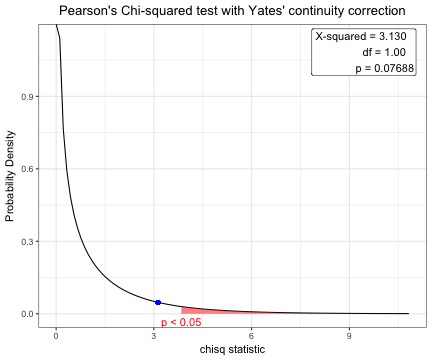## For one sample t-test

You can show the distribution of t-statistic and p-value in one sample t-test.


One Sample t-test

data:  acs\$age
t = 0.77978, df = 856, p-value = 0.4357
alternative hypothesis: true mean is not equal to 63
95 percent confidence interval:
62.52736 64.09574
sample estimates:
mean of x
63.31155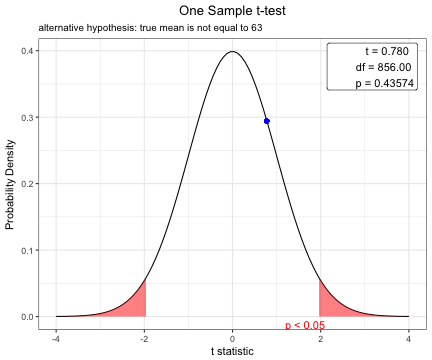## Student t-test to compare means for two independent samples

Before performing a t-test, you have to compare two variances.

### F test to compare two variances


F test to compare two variances

data:  age by DM
F = 1.2383, num df = 552, denom df = 303, p-value = 0.0376
alternative hypothesis: true ratio of variances is not equal to 1
95 percent confidence interval:
1.012416 1.505776
sample estimates:
ratio of variances
1.238288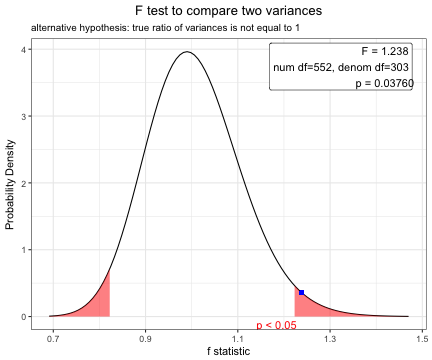### Use for Two Sample t-test for independence samples

Based on the result of var.test(), you can perform t.test with default option(var.equal=FALSE).


Welch Two Sample t-test

data:  age by DM
t = 0.58982, df = 682.36, p-value = 0.5555
alternative hypothesis: true difference in means is not equal to 0
95 percent confidence interval:
-1.112568  2.068014
sample estimates:
mean in group No mean in group Yes
63.48101          63.00329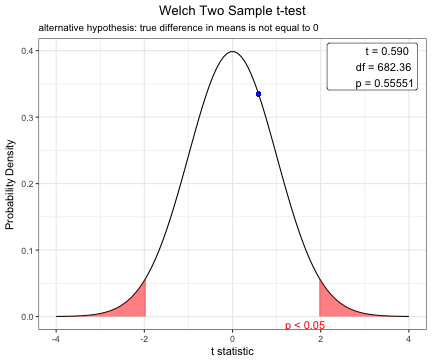## Student t-test using pooled variance

To compare means of body-mass index between male and female patients, perform F test first.


F test to compare two variances

data:  BMI by sex
F = 1.2078, num df = 254, denom df = 508, p-value = 0.07756
alternative hypothesis: true ratio of variances is not equal to 1
95 percent confidence interval:
0.9794315 1.5008098
sample estimates:
ratio of variances
1.207759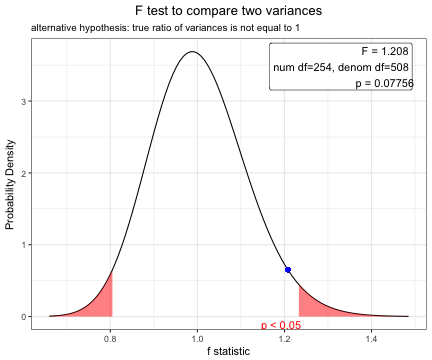Based on the result of F test, you can perform t-test using pooled variance.

Two Sample t-test

data:  BMI by sex
t = -0.50823, df = 762, p-value = 0.6114
alternative hypothesis: true difference in means is not equal to 0
95 percent confidence interval:
-0.6348532  0.3737344
sample estimates:
mean in group Female   mean in group Male
24.19492             24.32548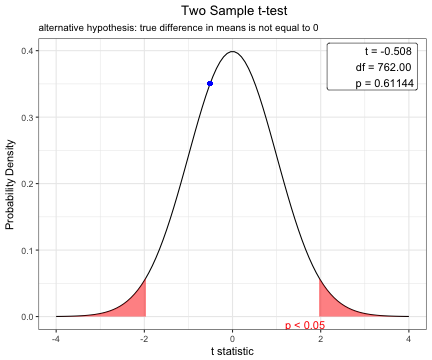## Paired t-test

You can show the distribution of t-statistic and p-value in paired t-test.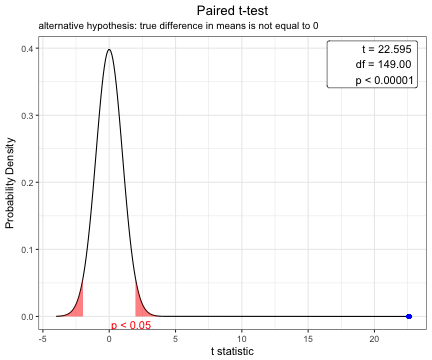## Options for t-test

You can change the options of t.test.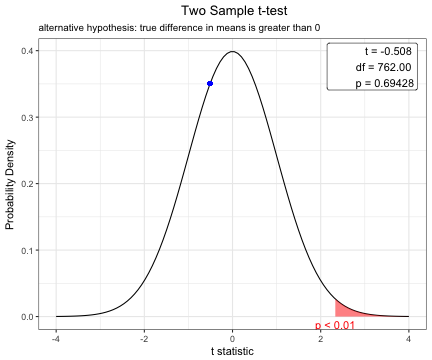# webr version 0.1.0

===================== (18-Apr-2018)

• new function rens.text() and cox.stuart.test() added

# Reference manual

install.packages("webr")

0.1.0 by Keon-Woong Moon, 2 years ago

https://github.com/cardiomoon/webr

Report a bug at https://github.com/cardiomoon/webr/issues

Browse source code at https://github.com/cran/webr

Authors: Keon-Woong Moon [aut, cre] , Tommaso Martino [ctb]

Documentation:   PDF Manual